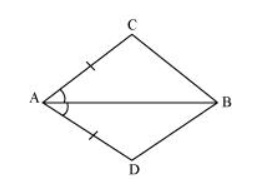# In quadrilateral $A C B D, A C=A D$ and $A B$ bisects $\angle A$ (See the given figure). Show that $\triangle A B C \cong \triangle A B D$. What can you say about $B C$ and $B D$ ?Solution:

In $\triangle \mathrm{ABC}$ and $\triangle \mathrm{ABD}$

$A C=A D$ (Given)

$\angle \mathrm{CAB}=\angle \mathrm{DAB}(\mathrm{AB}$ bisects $\angle \mathrm{A})$

$A B=A B$ (Common)

$\therefore \triangle \mathrm{ABC} \cong \triangle \mathrm{ABD}$ (By $S A S$ congruence rule)

$\therefore \mathrm{BC}=\mathrm{BD}(\mathrm{By} \mathrm{CPCT})$

Therefore, $B C$ and $B D$ are of equal lengths.